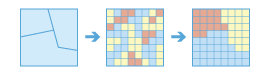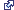Summarize Raster Within (Raster Analysis)

Summary

Calculates statistics on values of a raster within the zones of another dataset.

IllustrationUsage

• A zone is defined as all areas in the input with the same value. The areas do not have to be contiguous. Both raster and feature can be used for the zone input.

• This raster analysis portal tool is available when you are signed in to an ArcGIS Enterpriseportal that has an ArcGIS Image Serverconfigured for Raster Analysis. When the tool is invoked, ArcGIS Pro serves as a client and the processing happens in the servers federated with ArcGIS Enterprise. The portal tool accepts layers from your portal as input and creates output in your portal.

The input raster layer supports the following: a layer from the portal, a URI or URL to an image service, or the output from the Make Image Server Layer tool. The input feature layer can be a layer from the portal. It can also be a URI or URL to a feature service. This tool does not support local raster data or layers. While you can use local feature data and layers as input to this portal tool, best practice is to use layers from your portal as input.

• If the Input Zone Layer (inputZoneLayer in Python) is a raster, it must have an integer data type.

If the zone input is a feature, it will be converted to a raster internally using the cell size and cell alignment from the value raster.

• When the cell size of the Input Zone Layer (inputZoneLayer in Python) and the Input Raster Layer to Summarize (inputRasterLayertoSummarize in Python) are different, the output cell size will be the Maximum Of Inputs value, and the value raster will be used as the snap raster internally. If the cell size is the same but the cells are not aligned, the value raster will be used as the snap raster internally. Either of these cases will trigger an internal resampling before the zonal operation is performed.

When the zone and value inputs are both rasters of the same cell size and the cells are aligned, they will be used directly in the tool and will not be resampled internally during tool execution.

• If a particular zone from the Input Zone Layer does not overlap any cell centers of the input value raster, the zone will become NoData. In this case, determine an appropriate raster resolution that will retain the detail of your zone input and use this resolution as the cell size in the environment. Specifying a smaller cell size will generate a larger output raster. The higher resolution output will not necessarily be as high quality a result as it seems, since the additional detail does not actually exist in the Input Raster Layer to Summarize input.

• If the Input Zone Layer has overlapping polygons, the zonal analysis will not be performed for each individual polygon. Since the feature input is converted to a raster, each location can only have one value.

An alternative method is to process the zonal operation iteratively for each polygon zone and collate the results.

• If the Input Zone Layer is a point feature, it is possible to have more than one point contained within any particular cell of the input value raster. For such cells, the zone value is determined by the point with the lowest ObjectID field (for example, OID or FID).

• When specifying the Input Zone Layer, the default zone field will be the first available integer or text field. If no other valid fields exist, the ObjectID field (for example, OID or FID) will be the default.

• The Input Raster Layer to Summarize can be of either integer or floating data type. However, when it is floating data type, the options for calculating majority, median, minority, and variety will not be available. For majority and minority statistics, when there is a tie, the output for the zone is based on the lowest of the tied values.

• Supported multidimensional dataset types include multidimensional image service.

Syntax

SummarizeRasterWithin(inputZoneLayer, zoneField, inputRasterLayertoSummarize, outputName, {statisticType}, {ignoreMissingValues}, {processAsMultidimensional}, {percentileValue})
 Parameter Explanation Data Type inputZoneLayer The input that defines the zones.Both raster and feature data can be used for the zone input. Raster Layer; Feature Layer zoneField The field that defines each zone.It can be an integer or a string field of the zone dataset. String inputRasterLayertoSummarize The raster that contains the values on which to summarize a statistic. Raster Layer outputName The name of the output raster service.If the image service layer already exists, you will be prompted to provide another name. String statisticType(Optional) Specifies the statistic type to calculate.The available options when the raster to summarize is of integer data type are Average, Majority, Maximum, Median, Minimum, Minority, Percentile, Range, Standard deviation, Sum, and Variety.If the raster to summarize is of float data type, the options are Average, Maximum, Minimum, Range, Standard deviation, and Sum.MEAN —The average of all cells in the raster layer to be summarized that belong to the same zone as the output cell will be calculated. This is the default.MAJORITY —The value that occurs most often of all cells in the raster layer to be summarized that belong to the same zone as the output cell will be calculated.MAXIMUM —The largest value of all cells in the raster layer to be summarized that belong to the same zone as the output cell will be calculated.MEDIAN —The median value of all cells in the raster layer to be summarized that belong to the same zone as the output cell will be calculated.MINIMUM —The smallest value of all cells in the raster layer to be summarized that belong to the same zone as the output cell will be calculated.MINORITY —The value that occurs least often of all cells in the raster layer to be summarized that belong to the same zone as the output cell will be calculated.PERCENTILE —The percentile of all cells in the value raster that belong to the same zone as the output cell will be calculated. The 90th percentile is calculated by default. You can specify other values (from 0 to 100) using the Percentile Value parameter.RANGE —The difference between the largest and smallest value of all cells in the raster layer to be summarized that belong to the same zone as the output cell will be calculated.STD —The standard deviation of all cells in the raster layer to be summarized that belong to the same zone as the output cell will be calculated.SUM —The total value of all cells in the raster layer to be summarized that belong to the same zone as the output cell will be calculated.VARIETY —The number of unique values for all cells in the raster layer to be summarized that belong to the same zone as the output cell will be calculated. String ignoreMissingValues(Optional) Specifies whether missing values in the raster layer to summarize will be ignored in the results of the zones that they fall within. DATA —Within any particular zone, only cells that have a value in the raster layer being summarized will be used in determining the output value for that zone. Missing or NoData cells will be ignored in the statistic calculation. This is the default.NODATA —Within any particular zone, if any cells in the raster layer being summarized do not have a value, they will not be ignored and their existence indicates that there is insufficient information to perform statistical calculations for all the cells in that zone. Consequently, the entire zone will receive the NoData value on the output raster. Boolean processAsMultidimensional(Optional) Specifies how the input rasters will be processed if they are multidimensional.CURRENT_SLICE —Statistics will be calculated from the current slice of the input multidimensional dataset. This is the default.ALL_SLICES —Statistics will be calculated for all dimensions of the input multidimensional dataset. Boolean percentileValue(Optional) The percentile to calculate. The default is 90, indicating the 90th percentile.The values can range from 0 to 100. The 0th percentile is essentially equivalent to the minimum statistic, and the 100th percentile is equivalent to maximum. A value of 50 will produce essentially the same result as the median statistic.This option is only available if the statisticType parameter is set to PERCENTILE. Double

Derived Output

 Name Explanation Data Type outputRaster The output raster. Raster Layer

Code sample

SummarizeRasterWithin example 1 (Python window)

This example determines for each zone the average of cell values in the raster layer to be summarized.

import arcpy
arcpy.SummarizeRasterWithin_ra('https://MyPortal.esri.com/server/rest/services/Hosted/landuse/ImageServer',
'Landuse', 'https://MyPortal.esri.com/server/rest/services/Hosted/elevation/ImageServer',
'outRaster', 'MEAN', 'DATA')
SummarizeRasterWithin example 2 (stand-alone script)

This example determines for each zone the mean value in the raster layer to be summarized.

#-------------------------------------------------------------------------------
# Name: SummarizeRasterWithin_Ex_02.py
# Description: Calculates the maximum sea-surface temperature at different ecological zones.
# Requirements: ArcGIS Image Server

# Import system modules
import arcpy

# Set local variables
inputZoneLayer = 'https://MyPortal.esri.com/server/rest/services/Hosted/zones/ImageServer'
zoneField = 'temperature'
inputRasterLayertoSummarize = 'https://MyPortal.esri.com/server/rest/services/Hosted/seaSurfaceTemperature/ImageServer'
outputName = 'outSSTRaster'
statisticType = 'MAXIMUM'
ignoreMissingValues = ''
processAsMultidimensional = 'ALL_SLICES'

# Execute Multidimensional Summarize Raster Within operation
arcpy.SummarizeRasterWithin_ra(inputZoneLayer, zoneField, inputRasterLayertoSummarize,
outputName, statisticType, ignoreMissingValues)

Licensing information

• Basic: Requires ArcGIS Image Server
• Standard: Requires ArcGIS Image Server
• Advanced: Requires ArcGIS Image Server